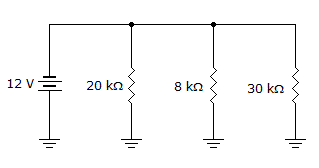# Electronics - Parallel Circuits - Discussion

### Discussion :: Parallel Circuits - Filling the Blanks (Q.No.4)

4.The total current in the given circuit is ___.

 [A]. 2.5 mA [B]. 0.6 mA [C]. 1.5 mA [D]. 0.4 mA

Explanation:

No answer description available for this question.

 Rajavarapu Narendhar said: (Jan 21, 2012) In the above 12v is same for each branch of parallel circuit I(total)=I1+I2+I3 So I1=12/20=0.6 mA I2=12/8=1.5mA I3=12/30=0.4mA Total current I(total)=0.6+1.5+0.4=2.5 mA.

 Shruti said: (Jul 1, 2016) Total resistance 1/R = 1/20 + 1/8 + 1/30, R = 120/25, I = V/R, 12*25/120 = 2.5.

 Clemet said: (Jul 5, 2018) In the above 12v is the same for each branch of the parallel circuit. I(total)=I1+I2+I3. So I1=12/20=0.6 mA. I2=12/8=1.5mA. I3=12/30=0.4mA. Total current I(total)=0.6+1.5+0.4=2.5 mA.